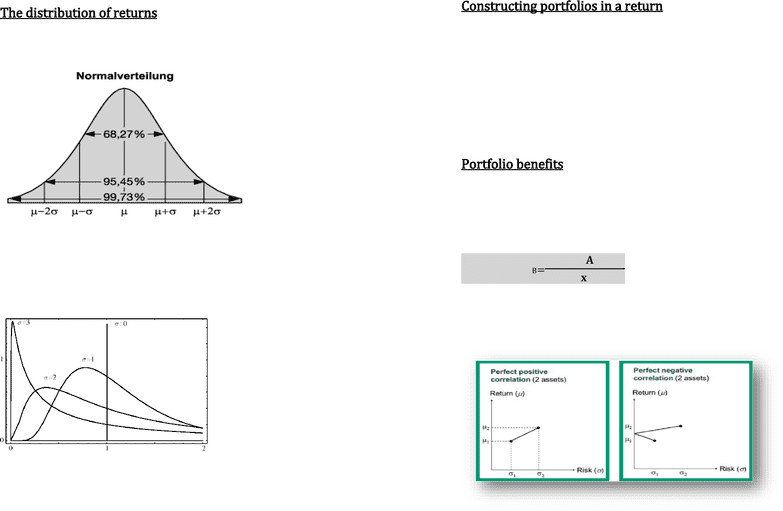Study Guides (390,000)
CA (150,000)
ECON (30)
Quiz

# ECON-1006EL Study Guide - Quiz Guide: Capital Asset Pricing Model, Log-Normal Distribution, Capital Asset

Department
Economics / Science Èconomique
Course Code
ECON-1006EL
Professor
Brian Mac Lean
Study Guide
Quiz

This preview shows page 1. to view the full 4 pages of the document.Portfolio theory and Capital asset pricing model
The distribution of returns
There are two forms of distributions, the normal distribution
and the
lognormal distribution.
In the short run the return distribution is close to the normal distribution.
As you see the normal distribution has no skewness and it only requires the
mean and variance to describe the whole distribution.
In the long run the returns are log-normally distributed.
The skewness results from the fact that returns >100% are possible, but
<100% not.
Constructing portfolios in a return
Standard deviation framework: Measures the deviation of an asset
from its mean. Therefore it’s a good measurement of risk.
The key question in the portfolio theory is to find for a given level of
risk the highest expected return which is possible.
Efficient portfolio
Portfolio benefits
In order to minimize risk we have to know how the assets in our
portfolio interact in between. So we have to measure the strength and
direction of the linear relationship between our (two) assets. Or in
other words: we have to calculate the correlation coefficient.
Corr A,B =𝞺A,B=𝐂𝐨𝐯[𝐫𝐀,𝐫𝐁]
𝐒𝐃[𝐫𝐀]𝐱 𝐒𝐃[𝐫𝐁]
Remember: the correlation coefficient is bound to a (-1,+1) interval.
Now we going to have a look on the effect on the portfolio risk.